# Introduction to PyTorch

PyTorch is a Python-based scientific computing package that is a replacement for NumPy to use the power of GPUs and TPUs and an automatic differentiation library useful to implement neural networks. The idea behind PyTorch was to make it as similar to Python’s NumPy as possible as doing so would allow smooth interaction between regular Python code, NumPy, and PyTorch leading to faster and easier implementation. PyTorch has rapidly garnered attention as the “TensorFlow killer” for being much more user-friendly and coherent with Python.

The most fundamental building block of any Deep Learning library is the tensor. Tensors are specialized data structures that are very similar to matrices. In PyTorch, tensors encode the inputs and outputs and the parameters of a model. Tensors are identical to NumPy’s n-dimensional arrays, except that they can run on GPUs to accelerate computing.

Tensors can be initialised in a lot of different ways. The below mentioned code initialises a tensor in three different ways:

``````import torch
import numpy as np

# declaring a (3x3) tensor containing random values using the tensor object
x = torch.Tensor(3, 3)
print(x)

# creating tensors directly from data
data = [[1, 2],[3, 4]]
x_data = torch.tensor(data)
print(x_data)

# Using numpy arrays to create tensors
np_array = np.array(data)
x_np = torch.from_numpy(np_array)
print(x_np)
``````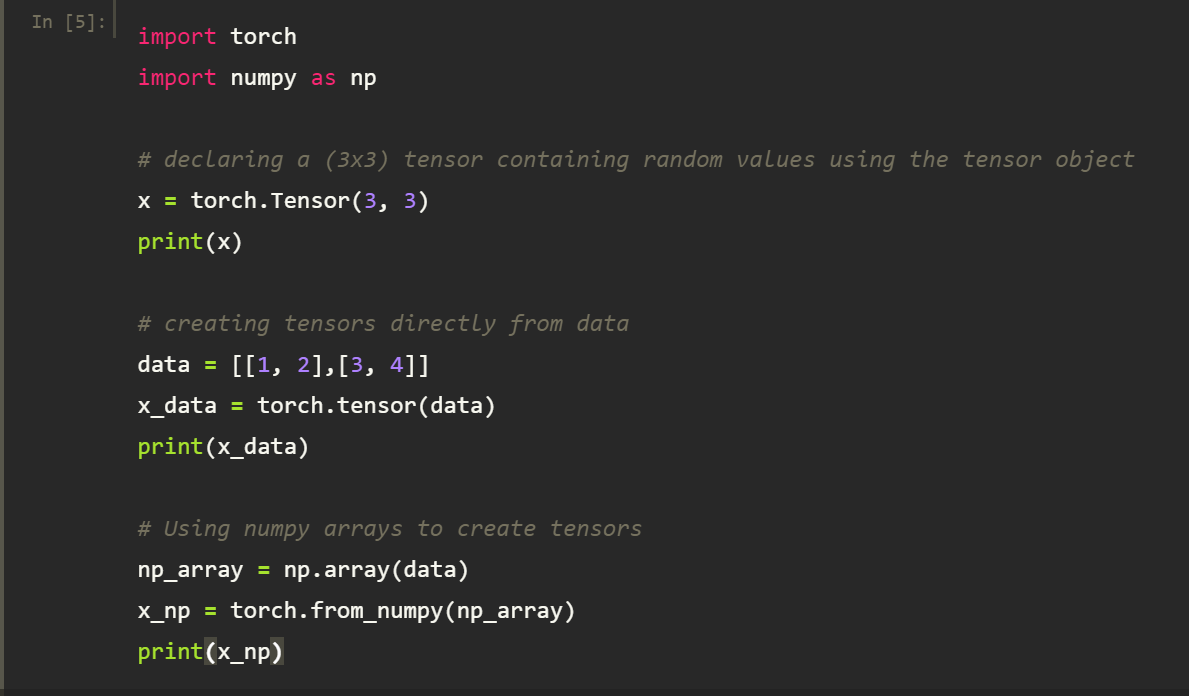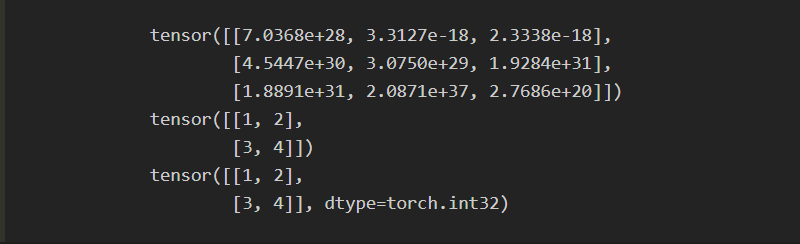Building a neural network using Pytorch

Pytorch defines neural networks as Python classes. The class representing the network extends the torch.nn.Module from the PyTorch library. The  __init__(), and the forward() functions are the Pytorch network module’s most essential functions. The __init__() is used to define any network layers of the model, whereas The forward() function sets up the model by stacking the layers together.

We will define an image classifier using neural networks and use the torchvision package from the Pytorch library specifically designed to deal with image data. We will use the CIFAR10 dataset, which contains images of size 3x32x32, i.e., 3-channel color images of 32×32 pixels in size depicting airplanes, automobiles, birds, cats, deers, dogs, frogs, horses, ships, and trucks.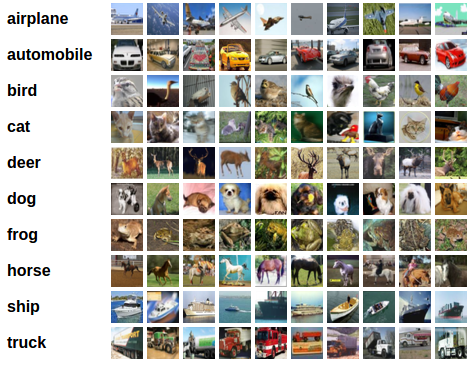source:CIFAR10

Use the following code to load up the CIFAR10 dataset, normalize it and import the important PyTorch libraries to be used in the training and testing phases.

``````# import all pytorch libraries and load the dataset
import torch
import torchvision
import torchvision.transforms as transforms
transform = transforms.Compose(
[transforms.ToTensor(),
transforms.Normalize((0.5, 0.5, 0.5), (0.5, 0.5, 0.5))])

trainset = torchvision.datasets.CIFAR10(root='./data', train=True,
shuffle=True, num_workers=2)

testset = torchvision.datasets.CIFAR10(root='./data', train=False,
shuffle=False, num_workers=2)

classes = ('plane', 'car', 'bird', 'cat', 'deer', 'dog', 'frog', 'horse', 'ship', 'truck')
``````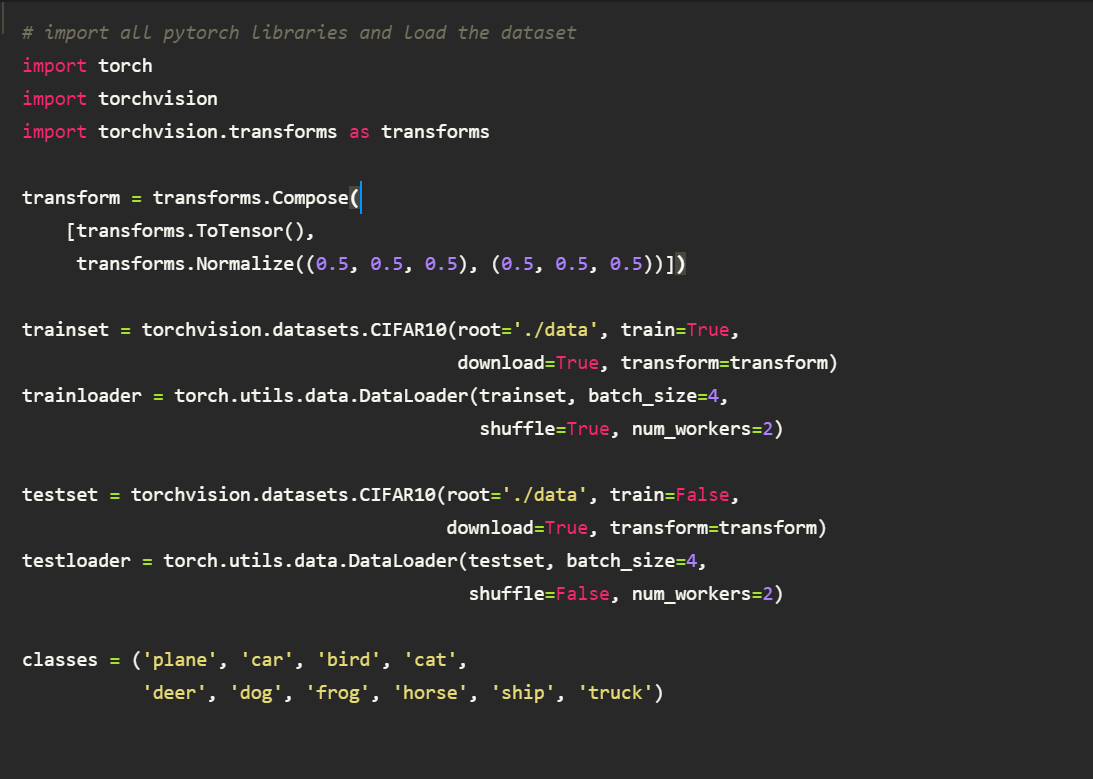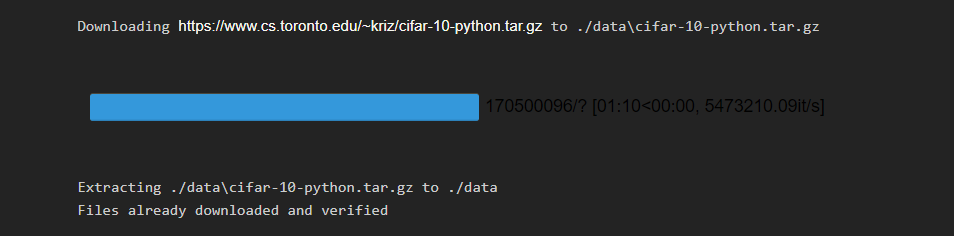The following code helps us view some images in the dataset and view the labels associated with them.

``````import matplotlib.pyplot as plt
import numpy as np

# functions to show an image
def imshow(img):
img = img / 2 + 0.5 # unnormalize
npimg = img.numpy()
plt.imshow(np.transpose(npimg, (1, 2, 0)))
plt.show()

# get some random training images
images, labels = dataiter.next()
# show images
imshow(torchvision.utils.make_grid(images))
# print labels
print(' '.join('%5s' % classes[labels[j]] for j in range(4)))
``````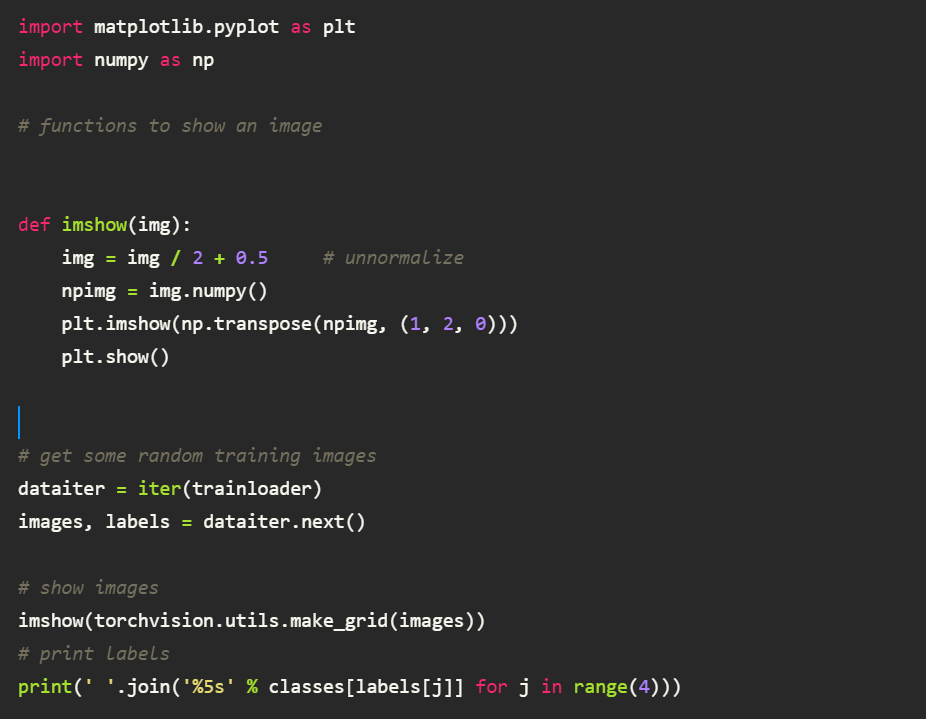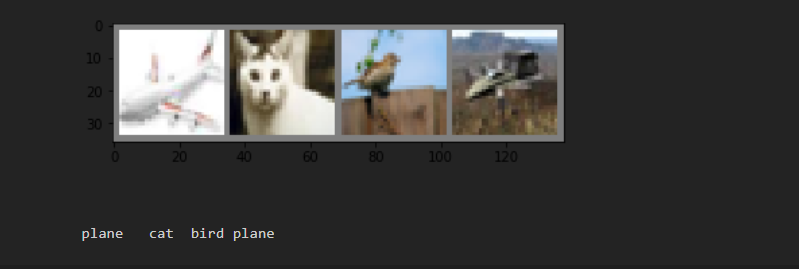Defining the Convolutional Neural Network

Use the below-mentioned code to implement a CNN network and train the model using the torch.nn package.

``````# import the neural network library from pytorch
import torch.nn as nn
import torch.nn.functional as F
# creating a neural network model
class Net(nn.Module):
def __init__(self):
super(Net, self).__init__()
self.conv1 = nn.Conv2d(3, 6, 5)
self.pool = nn.MaxPool2d(2, 2)
self.conv2 = nn.Conv2d(6, 16, 5)
self.fc1 = nn.Linear(16 * 5 * 5, 120)
self.fc2 = nn.Linear(120, 84)
self.fc3 = nn.Linear(84, 10)
# creating the feed-forward model
def forward(self, x):
x = self.pool(F.relu(self.conv1(x)))
x = self.pool(F.relu(self.conv2(x)))
x = x.view(-1, 16 * 5 * 5)
x = F.relu(self.fc1(x))
x = F.relu(self.fc2(x))
x = self.fc3(x)
return x
net = Net()

# using optimisers to tune the hyperparameters
import torch.optim as optim
criterion = nn.CrossEntropyLoss()
optimizer = optim.SGD(net.parameters(), lr=0.001, momentum=0.9)

for epoch in range(2): # loop over the dataset multiple times
running_loss = 0.0
for i, data in enumerate(trainloader, 0):
# get the inputs; data is a list of [inputs, labels]
inputs, labels = data
# forward + backward + optimize
outputs = net(inputs)
loss = criterion(outputs, labels)
loss.backward()
optimizer.step()

# print statistics
running_loss += loss.item()
if i % 2000 == 1999: # print every 2000 mini-batches
print('[%d, %5d] loss: %.3f' %
(epoch + 1, i + 1, running_loss / 2000))
running_loss = 0.0
print('Finished Training')
``````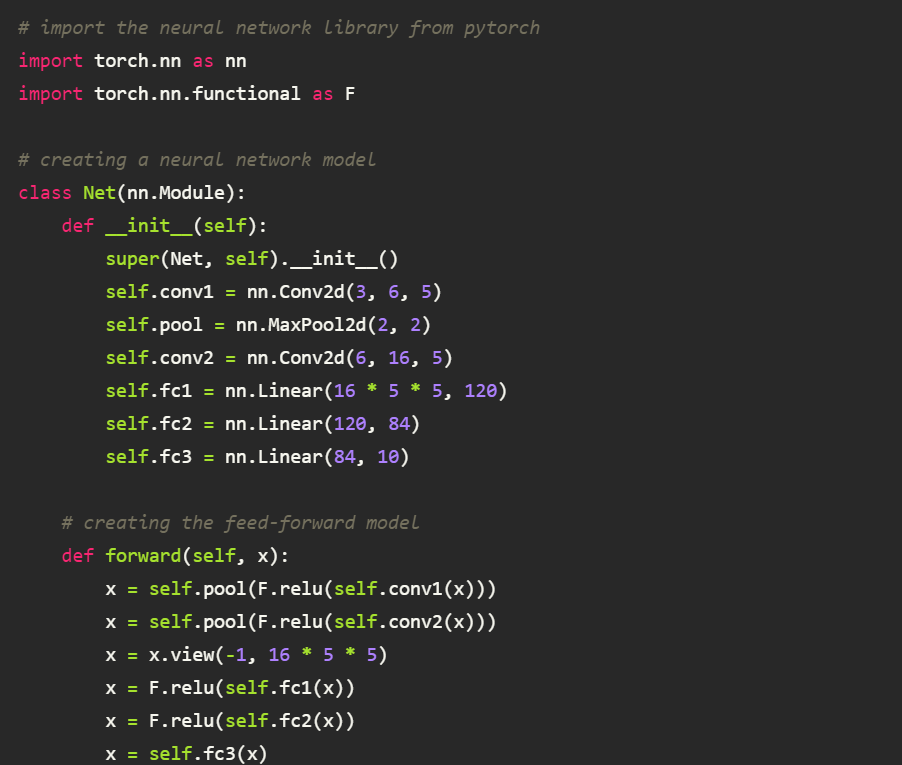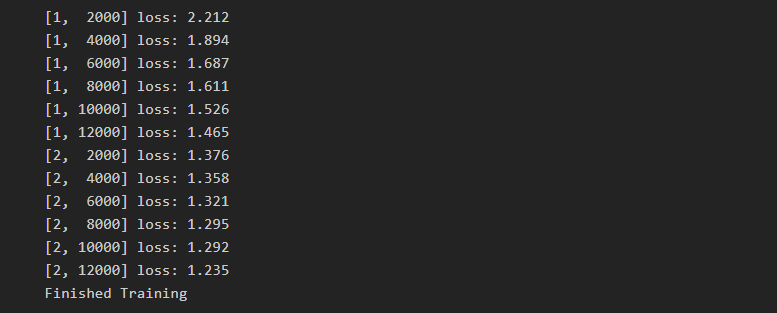Use the below-mentioned code to print the predictions from the model and find the model accuracy.

``````dataiter = iter(testloader)
images, labels = dataiter.next()
# print images
imshow(torchvision.utils.make_grid(images))
print('GroundTruth: ', ' '.join('%5s' % classes[labels[j]] for j in range(4)))

outputs = net(images)
_, predicted = torch.max(outputs, 1)
print('Predicted: ', ' '.join('%5s' % classes[predicted[j]]
for j in range(4)))

# calculating the accuracy of the model
correct = 0
total = 0
images, labels = data
outputs = net(images)
_, predicted = torch.max(outputs.data, 1)
total += labels.size(0)
correct += (predicted == labels).sum().item()
print('Accuracy of the network on the 10000 test images: %d %%' % (
100 * correct / total))
``````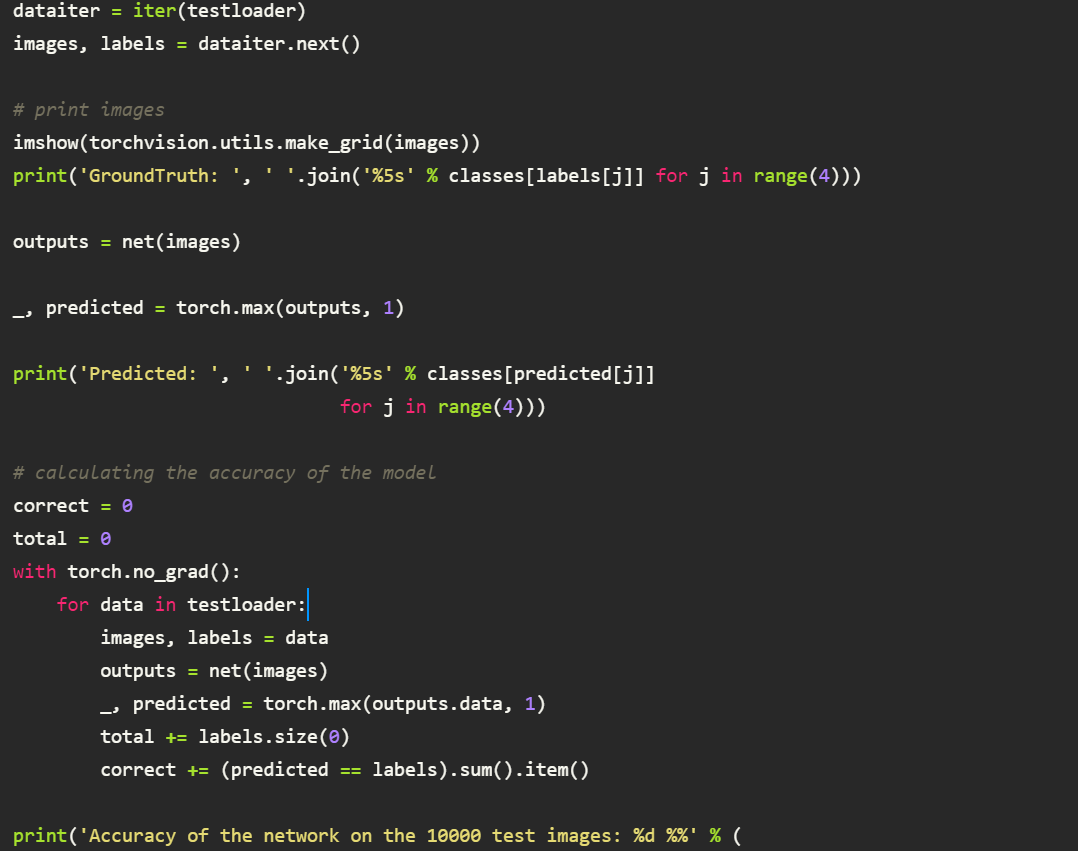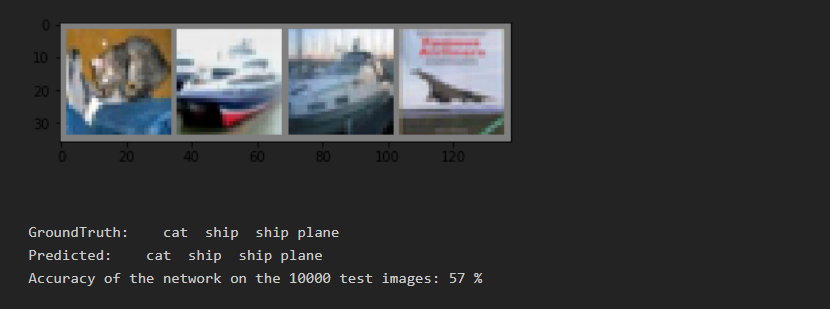Congratulations!!, you have made our First deep learning model using Pytorch.

Visit the official Pytorch website, which provides excellent documentation to all packages and functions. Happy learning!!

##### Nitish Kumar

Nitish is a computer science undergraduate with keen interest in the field of deep learning. He has done various projects related to deep learning and closely follows the new advancements taking place in the field.

🚀 The end of project management by humans (Sponsored)